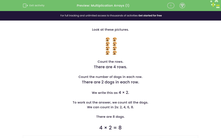# Understand Multiplication Arrays

In this worksheet, students will match pictorial arrays to multiplication statements.Key stage:  KS 1

Curriculum topic:   Number: Multiplication and Division

Curriculum subtopic:   Solve Multiplication/Division Problems

Difficulty level:#### Worksheet Overview

Look at these pictures:Count the rows.

There are 4 rows.

Count the number of dogs in each row.

There are 2 dogs in each row.

We write this as:   4 × 2

To work out the answer, we count all the dogs.

We can count in 2s: 2, 4, 6, 8.

There are 8 dogs.

4 × 2 = 8

Now look at these pictures:There are 5 rows.

There are 3 ladybirds in each row.

If we count all the ladybirds, we make a total of 15, but there is a quicker way to find out how many there are.

We write this as:   5 × 3

We could count in 3s or in 5s. Let's count in 5s because it is easier: 5, 10, 15

5 × 3 = 15

So, we know that there are 15 ladybirds in total.

Are you ready to have a go at some questions?### What is EdPlace?

We're your National Curriculum aligned online education content provider helping each child succeed in English, maths and science from year 1 to GCSE. With an EdPlace account you’ll be able to track and measure progress, helping each child achieve their best. We build confidence and attainment by personalising each child’s learning at a level that suits them.

Get started